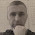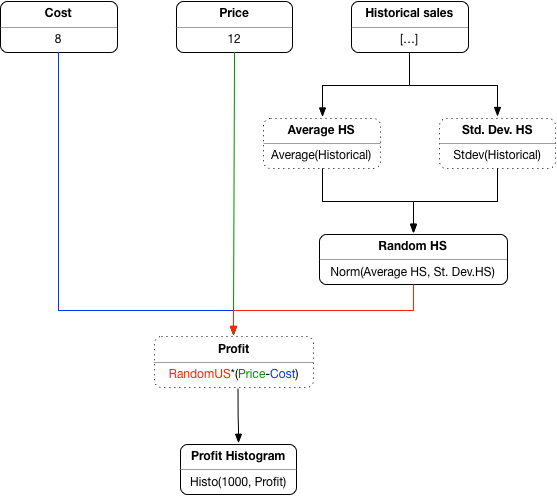## Tuesday, 17 July 2018

A programming language is truly "pure" if all computations produce a value -- Agda is an example of such a language. For all its claims to the contrary, Haskell is not a pure language. Computations in Haskell may produce exceptions (try taking the head of the empty list) or nothing at all (in the case of computations that run forever). I don't hold that against Haskell, I just dislike the propaganda. I don't hold that against Haskell because math itself is not pure in this sense, since particular operations could be undefined, for example division by zero. But could we say that Haskell is "as pure as maths"? In the (not very serious) post below I will argue to the contrary: Haskell is a lazy language whereas maths is an eager language, at least as commonly practiced.

In math we have functions, as we do in programming. The functions of programming can be either lazy or eager, depending on the programming language. Are the functions of math lazy or eager?

In programming, the issue is quite important because it has a significant impact on one of the key equations of the lambda calculus, the so-called beta-law, which says that we can substitute (i.e. inline) an argument for the parameter of the function.

(fun x. M)N ≡ M[N/x]

This law lies at the heart of all compiler optimisations.

The equation is always valid in call-by-name languages such as Algol. In lazy languages such as Haskell it still holds in the presence of some effects such as exceptions or non-termination, but it does not hold in the presence of stateful assignments. In an eager languages such as OCaml it is also invalidated in the presence of non-termination or exceptions. It is therefore common that in call-by-value languages the argument (N above) must be a value, i.e. a language constant, a variable, or a lambda expression, usually written as v.

(fun x.M)v ≡ M[v/x]

Of course, substitution is also an important rule of equational reasoning, which is a big part of mathematical calculations. But which rule do we use in math, the by-name, lazy or the by-value (eager) substitution? And how can we tell?

Lets look closer at when the beta law fails in an eager functional program. If the argument is not a value (N≠v) but it is equivalent to a value (Nv) then the rule holds. It only fails when the argument is not equivalent to a value, for example because it runs forever (it 'diverges'). An example of such a term is  Ω =(fun x.x x)(fun x.x x). Or, more mundanely, Ω = while true do skip. An example of failure of the beta law is

(fun x.k) Ω ≢ k[Ω/x]

where k is a language constant. If we run the left-hand side as a program it will first run the argument Ω, which will result in a nonterminating computation. On the other hand, the right-hand side is just the term consisting of the constant k because substitution happens at a syntactic level. So its value is, trivially, k. The two cannot be equivalent.

In maths we don't usually deal with functions such as Ω but we can still have expressions that don't denote a value, such as for example 1/m when m is 0. Both Ω and 1/m are 'undefined' in this sense, that they don't denote a value.

The following example will test whether maths is lazy or eager. Consider the constant function

c(m) = 1

The question is whether the equation

mc(1/m) = 0

has a solution (m=0) or not.

I have not run any referenda on the matter, but from my own understanding of mathematical practice and from pub chats with mathematician pals I would say that m=0 is not considered a valid solution for the above. A function such as the constant function above is defined over values and not over terms.

Perhaps it could be otherwise, but it ain't. Meanwhile, in Haskell

let c(x) = 1 in let m = 0 in m * (c (1/m))

will always evaluate to 0 (or rather 0.0 to be more precise).

So Haskell is impure. And not even mathematically so.

1.Hooray! The bullshit field surrounding Haskell is almost impenetrable. I've made my own efforts, and I applaud yours, to penetrate it.

There are a zillion possible topics, but one is to debunk the myth that Haskell has any special relationship to category theory not shared by, say, ML --- namely none at all. Haskell "monads" are not monads.

And Haskell is a dialect of Algol, as I explain in PFPL. The modal distinction between expressions and commands goes back to 1960.

2.